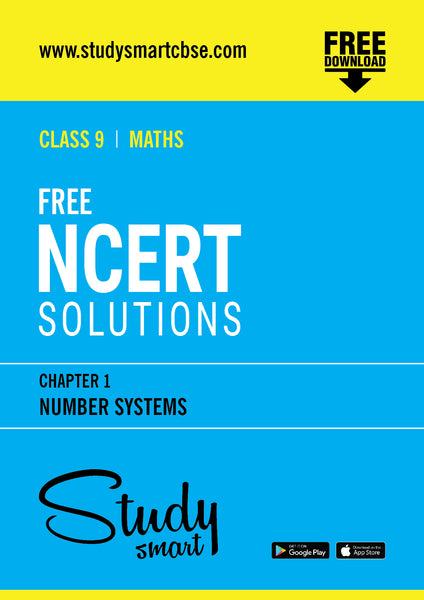# FREE NCERT Solutions Class 9th Maths Chapter 1 Number Systems

This will be sent as a link to your registered email id

#### Description:

REAL NUMBERS (18 Periods)

1. Review of representation of natural numbers, integers, rational numbers o the number line. Representation of terminating / non-terminating recurrin decimals on the number line through successive magnifcation. Rationa numbers as recurring/ terminating decimals. Operations on real numbers

2. Examples of non-recurring/non-terminating decimals. Existence of nonrational numbers (irrational numbers) such as √2, √3

3. Definition of nth root of a real number.

4. Existence of √x for a given positive real number x and its representation on the number line with geometric proof.

5. Rationalization (with precise meaning) of real numbers of the type 1/(a+b√x) &,1/(√x+√y)(and their combinations) where x and y are natural number and a and b are integers.

6. Recall of laws of exponents with integral powers. Rational exponents with positive real bases (to be done by particular cases, allowing learner to arrive at the general laws.)